Courses

# 28 Year NEET Questions: Ray Optics and Optical Instruments- 2

## 30 Questions MCQ Test Physics 28 Years Past year papers for NEET/AIPMT Class 12 | 28 Year NEET Questions: Ray Optics and Optical Instruments- 2

Description
This mock test of 28 Year NEET Questions: Ray Optics and Optical Instruments- 2 for NEET helps you for every NEET entrance exam. This contains 30 Multiple Choice Questions for NEET 28 Year NEET Questions: Ray Optics and Optical Instruments- 2 (mcq) to study with solutions a complete question bank. The solved questions answers in this 28 Year NEET Questions: Ray Optics and Optical Instruments- 2 quiz give you a good mix of easy questions and tough questions. NEET students definitely take this 28 Year NEET Questions: Ray Optics and Optical Instruments- 2 exercise for a better result in the exam. You can find other 28 Year NEET Questions: Ray Optics and Optical Instruments- 2 extra questions, long questions & short questions for NEET on EduRev as well by searching above.
QUESTION: 1

### Focal length of a convex lens of refractive index1.5 is 2 cm. Focal length of the lens whenimmersed in a liquid of refractive index of 1.25 will be 

Solution: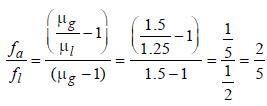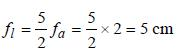QUESTION: 2

### Ray optics is valid, when characteristicdimensions are 

Solution:

Characteristic dimensions must be much
larger than the wavelength of light.

QUESTION: 3

### A ray is incident at an angle of incidence i on one surface of a prism of small angle A and emerges normally from the opposite surface. If the refractive index of the material of prism is μ, the angle of incidence i is nearly equal to    

Solution:

As refracted ray emerges normally from
oppostite surface, r2 = 0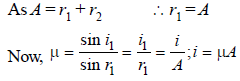QUESTION: 4

A beam of monochromatic light is refracted fromvacuum into a medium of refractive index 1.5,the wavelength of refracted light will be (1991]

Solution: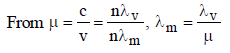Here, c = velocity of light in medium and v =
velocity of light in vacuum;
m = refractive index of the medium.
Hence, wavelength in medium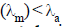(∴ μ > 1, given)

So, the required wavelength decreases.
ALTERNATIVELY,
c =vλ . On refraction, the frequency, v do
not change. When light is refracted from
vacuum to a medium, the velocity, c
decreases. Therefore,  λ also decreases

QUESTION: 5

Green light of wavelength 5460 Å is incident onan air-glass interface. If the refractive index ofglass is 1.5, the wave length of light in glasswould be (c = 3 × 108 ms–1) 

Solution: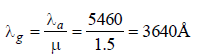QUESTION: 6

There is a prism with refractive index equal to √2 and the refracting angle equal to 30°. One of the refracting surfaces of the prism is polished. A beam of monochromatic light will retrace its path if its angle of incidence over the refracting surface of the prism is 

Solution:It is clear from the figure that the ray will
retrace the path when the refracted ray QR is
incident normally on the polished surface AC.
Thus, angle of refraction r = 30°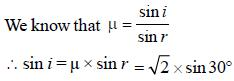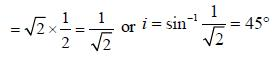QUESTION: 7

Time taken by sunlight to pass through a window of thickness 4 mm whose refractive index is 3/2 is 

Solution: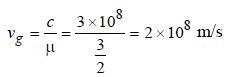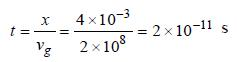QUESTION: 8

A point source of light is placed 4 m below thesurface of water of refractive index 5/3. Theminimum diameter of a disc, which should beplaced over the source, on the surface of waterto cut off all light coming out of water is 

Solution: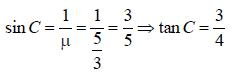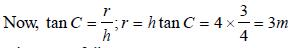QUESTION: 9

Angle of deviation (δ) by a prism (refractive index = μ and supposing the angle of prism A to be small) can be given by 

Solution:

When the angle of prism is small, δ = (μ – 1) A

QUESTION: 10

Focal length of a convex lens will be maximumfor 

Solution:

For red light, focal length of lens is maximum
because f ∝ λ  and λ is maximum for red
light.

QUESTION: 11

An achromatic combination of lenses is formedby joining 

Solution:

If two or more lenses are combined together
in such a way that this combination
produces images of different colours at the
same point and of the same size, then this
property is called ‘achromatism’. Concave
and convex type of lenses are used for this
combination.

QUESTION: 12

The hypermetropia is a 

Solution:

A person suffering from hyper metropia can
see objects beyond a particular point called
the near point. If the object lies at a point
nearer than this point, then image is not
formed at the retina. This is also known as
long-sight defect.

QUESTION: 13

If two mirrors are kept inclined at 60° to eachother and a body is placed at the middle, thentotal number of images formed is 

Solution:

Angle between two mirrors (θ) = 60º. Number
of images formed by the inclined mirror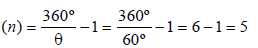QUESTION: 14

A lens is placed between a source of light and a wall. It forms images of area A1 and A2 on the wall for its two different positions. The area of the source of light is 

Solution:

Size of images = A1 and A2. From the
displacement method, the area of the source
of light =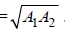QUESTION: 15

A convex lens of focal length 80 cm and aconcave lens of focal length 50 cm are combinedtogether. What will be their resulting power?      

Solution:

We know that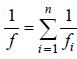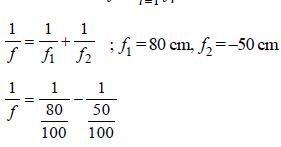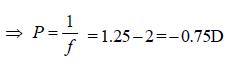QUESTION: 16

If fV and fR are the focal lengths of a convex lensfor violet and red light respectively and FV and FR are the focal lengths of concave lens for violetand red light respectively, then we have 

Solution: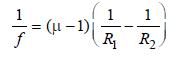According to Cauchy relation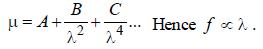Hence, red light having maximum wavelength
has maximum focal length.

∴ fv < fr and also Fv > Fr as focal length is
negative for a concave lens.

QUESTION: 17

Light travels through a glass plate of thickness t and refractive index μ. If c is the speed of light in vacuum, the time taken by light to travel this thickness of glass is

Solution:

Total thickness = t; Refrative index = μ

Speed of light in Glass plate = c/μ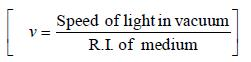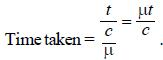[where, t = thickness of glass plate]

QUESTION: 18

One face of a rectangular glass plate 6 cm thick issilvered. An object held 8 cm in front of the firstface forms an image 12 cm behind the silveredface. The refractive index of the glass is 

Solution:

Thickness of glass plate (t) = 6 cm;
Distance of the object (u) = 8 cm.
And distance of the image (v) = 12 cm.
Let x = Apparent position of the silvered
surface in cm.
Since the image is formed due to relfection at
the silvered face and by the property of mirror
image
Distance of object from the mirror = Distance
of image from the mirror
or, x + 8 = 12 + 6 – x ⇒ x = 5 cm.
Therefore, refractive index of glass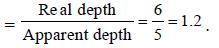QUESTION: 19

The focal length of converging lens is measuredfor violet, green and red colours. It is respectively fv, fg, fr. We will get 

Solution:

According to Cauchy relation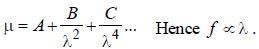∴ Red light has wavelength greater than
violet light. Therefore focal length of lens
for red is greater than for violet. (fr > fv)

QUESTION: 20

An astronomical telescope has a length of 44 cmand tenfold magnification. The focal length ofthe objective lens is 

Solution:

Given : Length of astronomical telescope
(f0 + fe) = 44 cm and magnification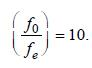From the given magnification, we find that f0
= 10fe. Therefore, 10fe + fe = 44 or 11fe = 44 or
fe = 4. And focal length of the objective
(f0) = 44 – fe = 44 – 4 = 40 cm.

QUESTION: 21

An electromagnetic radiation of frequency n, wavelength λ, travelling with velocity v in air enters in a glass slab of refractive index (ν). The frequency, wavelength and velocity of light in the glass slab will be respectively 

Solution:

When electromagnetic wave enters in other
medium, frequency reamains unchanged
while wavelength and velocity become 1/μ times.

So, For e.m. wave entering from air to glass
slab (μ), frequency remains n,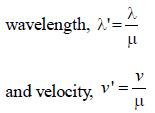QUESTION: 22

A luminous object is placed at a distance of 30 cm from the convex lens of focal length 20 cm. On theother side of the lens, at what distance from thelens a convex mirror of radius of curvature 10 cmbe placed in order to have an upright image of theobject coincident with it? 

Solution: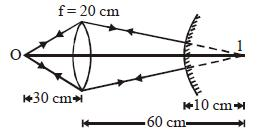For the lens,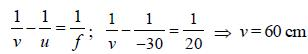Coincidence is possible when the image is
formed at the centre of curvature of the
mirror. Only then the rays refracting through
the lens will fall normally on the convex mirror
and retrace their path to form the image at O.
So, the distance between lens and mirror
= 60 – 10 = 50 cm.

QUESTION: 23

Light enters at an angle of incidence in atransparent rod of refractive index n. For whatvalue of the refractive index of the material ofthe rod the light once entered into it will notleave it through its lateral face whatsoever bethe value of angle of incidence? 

Solution:

Let a ray of light enter at A and the refracted
beam is AB. This is incident at an angle θ.
For no refraction at the lateral face, θ > C
or, sin θ > sin C But θ+ r =90°⇒θ= (90° – r)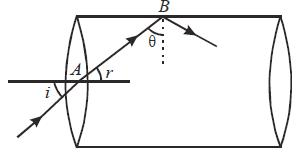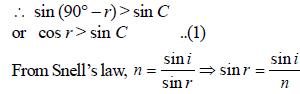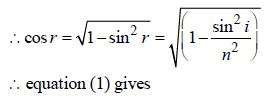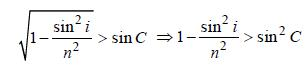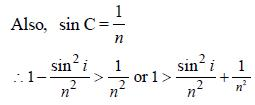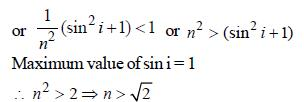QUESTION: 24

A plano-convex lens is made of material ofrefractive index 1.6. The radius of curvature ofthe curved surface is 60 cm. The focal length ofthe lens is 

Solution: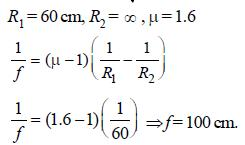QUESTION: 25

Wavelength of light of frequency 100 Hz 

Solution: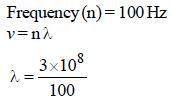QUESTION: 26

The refractive index of the material of the prismis √3 ; then the angle of minimum deviation ofthe prism is 

Solution:

Angle of minimum deviation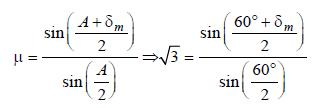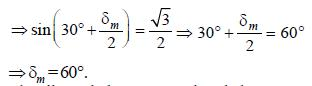QUESTION: 27

The radius of curvature of a thin plano-convexlens is 10 cm (of curved surface) and the refractiveindex is 1.5. If the plane surface is silvered, thenit behaves like a concave mirror of focal length   

Solution:

The silvered plano convex lens behaves as a
concave mirror; whose focal length is given by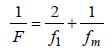If plane surface is silvered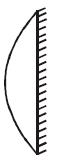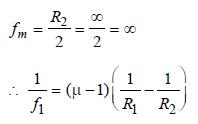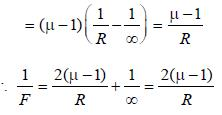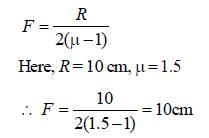QUESTION: 28

A person is six feet tall. How tall must a verticalmirror be if he is able to see his entire length?

Solution:

To see his full image in a plane mirror a person
requires a mirror of at least half of his height.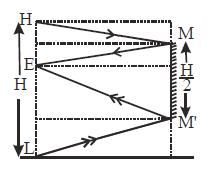QUESTION: 29

Rainbow is formed due to a combination of   

Solution:

Rainbow is formed due to combination of
total internal reflection and dispersion.

QUESTION: 30

An air bubble in a glass slab (μ = 1.5) is 5 cmdeep when viewed from one face and 2 cm deepwhen viewed from the opposite face. Thethickness of the slab is 

Solution: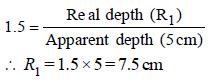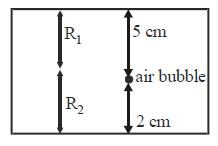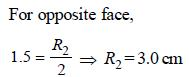∴ Thickness of the slab = R1 + R2
= 7.5 + 3 = 10.5 cm